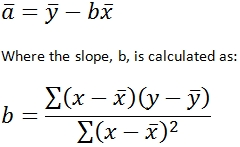# SQL Server INTERCEPT function

INTERCEPT_q

Updated: 9 August 2010

Use INTERCEPT_q to calculate the point at which a line will intersect the y-axis by using existing x-values and y-values. The y-intercept is the value of the point at which intersects the line at x = 0. In linear equations that are in the slope intercept from of y = mx + b, the value of b is the y-intercept. The equation for intercept is:Syntax
SELECT [wctStatistics].[wct].[INTERCEPT_q] (
<@Known_y_Known_x_RangeQuery, nvarchar(4000),>)
Arguments

@Known_y_Known_x_RangeQuery
the select statement, as text, used to determine the known y- and x-values to be used in the INTERCEPT_q function.
Return Types
float
Remarks
·         If the number of y-data points is not equal to the number of x-data points, INTERCEPT will return an error.
·         No GROUP BY is required for this function even though it produces aggregated results.
Examples
CREATE TABLE #i1(
[y] [float] NOT NULL,
[x] [float] NOT NULL
)
INSERT INTO #i1 VALUES (-4.5,-1)
INSERT INTO #i1 VALUES (0,2)
INSERT INTO #i1 VALUES (-9,-4)
INSERT INTO #i1 VALUES (4.5,5)
INSERT INTO #i1 VALUES (-13.5,-7)
SELECT wct.INTERCEPT_q('SELECT y, x from #i1')

This produces the following result

----------------------
-3

(1 row(s) affected)Copyright 2008-2021 Westclintech LLC         Privacy Policy        Terms of Service